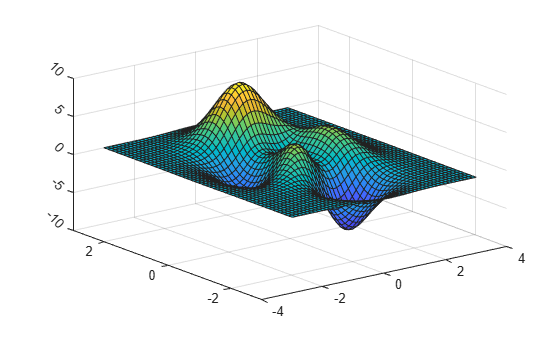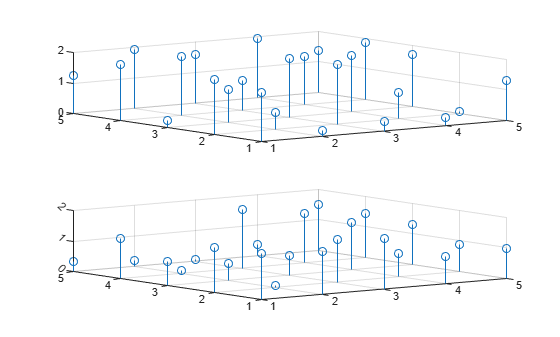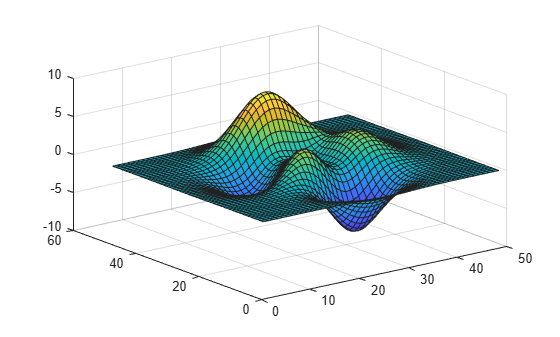# ztickangle

Rotate z-axis tick labels

## Syntax

``ztickangle(angle)``
``ztickangle(ax,angle)``
``ang = ztickangle``
``ang = ztickangle(ax)``

## Description

example

````ztickangle(angle)` rotates the z-axis tick labels for the current axes to the specified angle in degrees, where `0` is horizontal. Specify a positive value for counterclockwise rotation or a negative value for clockwise rotation.```

example

````ztickangle(ax,angle)` rotates the tick labels for the axes specified by `ax` instead of the current axes.```

example

````ang = ztickangle` returns the rotation angle for the z-axis tick labels of the current axes as a scalar value in degrees. Positive values indicate counterclockwise rotation. Negative values indicate clockwise rotation.```
````ang = ztickangle(ax)` uses the axes specified by `ax` instead of the current axes.```

## Examples

collapse all

Create a surface chart and rotate the z-axis tick labels 45 degrees clockwise.

```[x,y,z] = peaks; surf(x,y,z) ztickangle(-45)```Starting in R2019b, you can display a tiling of plots using the `tiledlayout` and `nexttile` functions. Call the `tiledlayout` function to create a 2-by-1 tiled chart layout. Call the `nexttile` function to create the axes objects `ax1` and `ax2`. Plot into each of the axes. Then rotate the z-axis tick labels for the lower plot by specifying `ax2` as the first input argument to `ztickangle`.

```tiledlayout(2,1) ax1 = nexttile; stem3(ax1,2*rand(5)) ax2 = nexttile; stem3(ax2,2*rand(5)) ztickangle(ax2,-45)```Create a surface chart. Then, query the rotation angle for the z-axis tick labels. By default, the labels are not rotated.

```[x,y,z] = peaks; surf(peaks)````ang = ztickangle`
```ang = 0 ```

## Input Arguments

collapse all

Rotation of tick labels, specified as a scalar value in degrees, where 0 is horizontal.

Example: `ztickangle(90)`

Target axes, specified as an `Axes` object or an array of `Axes` objects.

If you do not specify this argument, then `ztickangle` modifies the current axes.

## Algorithms

The `ztickangle` function sets and queries the `ZTickLabelRotation` property of the `Axes` object.

## Version History

Introduced in R2016b